This guide introduces the basic usage of tsdf. For more details, see the documentation for individual functions.

## Installation

Type the following command in R console :

install.packages("tsdf")

#check if devtools is installed
if(!"devtools" %in% rownames(installed.packages())){
install.packages(devtools)
}
devtools::install_github("wguo1990/tsdf")

Then the R package will be downloaded and installed to the default directories. Run the following command to load the package :

library(tsdf)

We will briefly go over main functions and their basic usage in the following sections.

## Zhong’s 2-/3-stage Phase II designs

To calculate Zhong’s two-/three-stage design, users need to provide : left-side type I error(alpha1), right-side type I error(alpha2), type II error(beta), minimal response rate(pc) and unacceptable response rate(pt, alternative). The minimal response rate can be either single point or an interval. stage option specify 2 or 3 stage design. Run the following command to obtain a 2-stage design

# type I errors
alpha1 <- 0.15
alpha2 <- 0.10
# type II error
beta <- 0.15
# response rate
pc <- 0.25
# alternative
pt <- pc + 0.20
# 2-stage design
out <- opt.design(alpha1, alpha2, beta, pc, pt, stage = 2)

out is an object of class opt.design. S3 method print is available for opt.design class. To extract information, run

print(out)
##
##  Zhong's 2 stage Phase II design
##
## Minimal response rate:  0.45
## Postulate response rate:
## Left-side type 1 error:  0.15
## Right-side type 1 error:  0.1
## Type 2 error:  0.15
##
## Notation:
## Left-side rejection region at stage i is response <= ri
## Right-side rejection region at stage 2 is response > s
## Sample size used at stage i is ni
##
## Optimal design :
## r1 r2 s1 s2 n1 n2
##  0  4  9 11  9 24
## True errors :
## alpha11 alpha12 alpha21 alpha22    beta
##  0.0751  0.1152  0.0000  0.0982  0.1217

Alpha-spending method is added to two-/three-stage designs. opt.design supports Hwang-Shih-DeCani spending function where sf.param specifies the parameter. For two-stage design, the default value is set to be NULL which means alpha-spending is not used. For three-stage design, the default is 4. User can change this parameter to set the alpha spent at first 1 or 2 stage. For example, to use a Pocock-like spending function, let sf.param = 1, then run

opt.design(alpha1, alpha2, beta, pc, pt, stage = 2, sf.param = 1)

## Decision table for Phase I dose-finding

dec.table function is used to generate decision table for a three-stage dose-finding design. alpha.l (left side), alpha.r (right side), alpha.u (right side type I error for “DU”, usually less than alpha.r) are three type I errors control the boundary of decisions. pt is the target toxicity level. pt is either a single or an interval value. This is also a group sequential design, so alpha-spending method is added. The sf.param option is same as in opt.design. The default value is set to be 4. We call dec.table function as follows

# sample size
n <- rep(3, 3)
# type I errors
alpha.l <- 0.6
alpha.r <- 0.4
alpha.u <- 0.2
# target toxicity
pt <- 0.3
# call dec.table
out <- dec.table(alpha.l, alpha.r, alpha.u, pt, n)

out is an object of class dec.table that contains all the relevant information including decision table, real type errors and input parameter. However, we do not recommend users to extract the components from this object directly. plot and print S3 method are available for dec.table class.

We can view the decision table using print function :

print(out)
##   3  6  9
## 0 E  E  E
## 1 S  E  E
## 2 D  S  E
## 3 DU D  S
## 4 0  DU D
## 5 0  DU DU
## 6 0  DU DU
## 7 0  0  DU
## 8 0  0  DU
## 9 0  0  DU
##
##    Row : Number of DLTs
## Column : Number of subjects
##      E : Escalate to the next higher dose
##      S : Stay at the same dose
##      D : De-escalate to the previous lower dose
##     DU : De-escalate and never use this dose again
##
## Type 1 error :
##   alpha11   alpha12   alpha13   alpha21   alpha22   alpha23
## 0.3430000 0.4942630 0.5609700 0.2160000 0.3112560 0.3532639
## Type 2 error :  0.1690468
## Right-side type 1 error :
##   alpha11   alpha12   alpha13
## 0.0270000 0.0797310 0.1264898

or visualize the decision table by executing the plot function :

plot(out)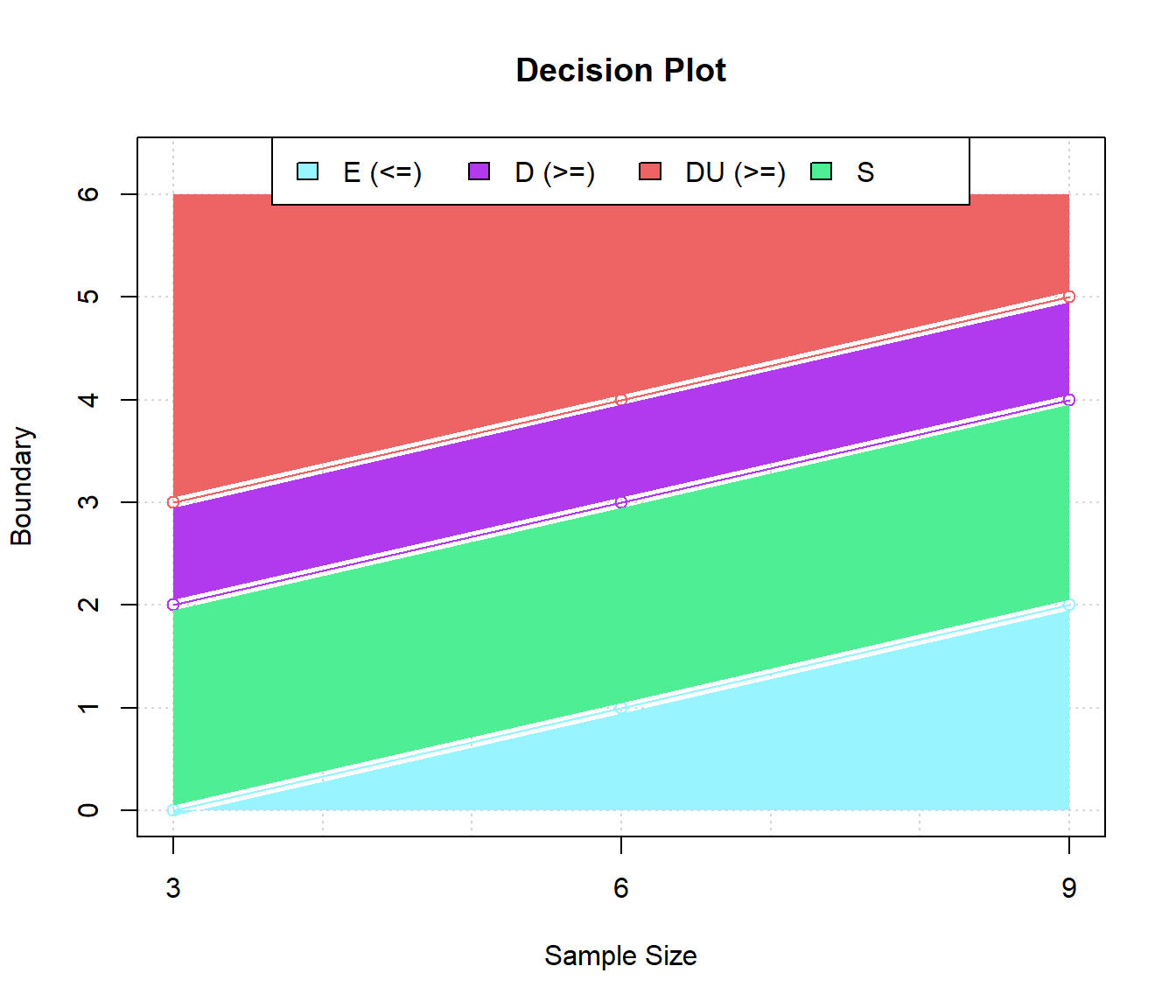## Dose-finding simulations

There are two functions that allow users to run dose-finding simulations using a user supplied decision table: dec.sim and sl.sim. To run simulations, users need to provide a decision table either from dec.table function or a user-supplied table (see details in next section), true probability of toxicity at each dose level, starting dose (default to lowest dose level) and number of simulated trials (default to 1000).

dec.sim runs one scenario simulation which is usually used for an initial test and sl.sim runs a list of scenarios from .csv or .txt files (See next section Data Format for details). Let’s see an example :

# true toxicity
truep <- c(0.3, 0.45, 0.5, 0.6)
# generate a decision table
dt <- dec.table(0.6,0.4,0.2,0.3,c(3,3,3))
# run simulation
out1 <- dec.sim(truep, dt$table, start.level = 2, nsim = 1000) The following command loads a sample scenarios list in tsdf package : test.file <- system.file("extdata", "testS.csv", package = "tsdf") Run simulations using sl.sim : out2 <- sl.sim(dt$table, test.file)

out1 and out2 are both dec.sim class object. out2 is also a class of sl.sim when the scenarios is more than 2. S3 method summary and plot are availiale for this class. For example, a summary of commonly used statistics is reported if we use the summary function :

# target toxicity
pt <- c(0.3, 0.4)
summary(out2, pt)
## What does each column represent ?
##
## Level : Dose level
## Truth : True toxicity probability
## MTD   : The probability of selecting current dose level as the MTD
## Over  : The probability of selecting current dose level as over the MTD
## DLT   : The average number of subjects experienced DLT at current dose level
## NP    : The average number of subjects treated at current dose level
##
## Scenario  1
##
## Simulation results are based on 1000 simulated trials
## Starting dose level is 1 ; MTD is dose 1
## Target toxicity probability = 0.3
## Average number of subjects = 10.557
## Probability of selecting the true MTD = 0.478
## Probability of subjects treated at or below the true MTD = 0.627451
##
##   Level Truth   MTD  Over   DLT    NP
## 1     1  0.30 0.478 0.375 1.998 6.624
## 2     2  0.45 0.121 0.853 1.416 3.081
## 3     3  0.50 0.024 0.974 0.367 0.726
## 4     4  0.60 0.000 1.000 0.073 0.126
##
## Scenario  2
##
## Simulation results are based on 2000 simulated trials
## Starting dose level is 2 ; MTD is dose 3
## Target toxicity probability = 0.4
## Average number of subjects = 15.5385
## Probability of selecting the true MTD = 0.672
## Probability of subjects treated at or below the true MTD = 0.8201564
##
##   Level Truth    MTD   Over    DLT     NP
## 1     1  0.01 0.0395 0.0000 0.0025 0.3405
## 2     2  0.10 0.2550 0.0395 0.5040 5.0580
## 3     3  0.25 0.6720 0.2945 1.8155 7.3455
## 4     4  0.65 0.0315 0.9665 1.7060 2.6580
## 5     5  0.70 0.0000 1.0000 0.0945 0.1365

There are four different type of plots for dec.sim object where option s indicates the number of scenario you want to plot and pt is the target toxicity for each scenario, see more details in plot.dec.sim R documentation:

# input information (true toxicity)
plot(out2, s = 2, pt = c(0.3, 0.4), type="s")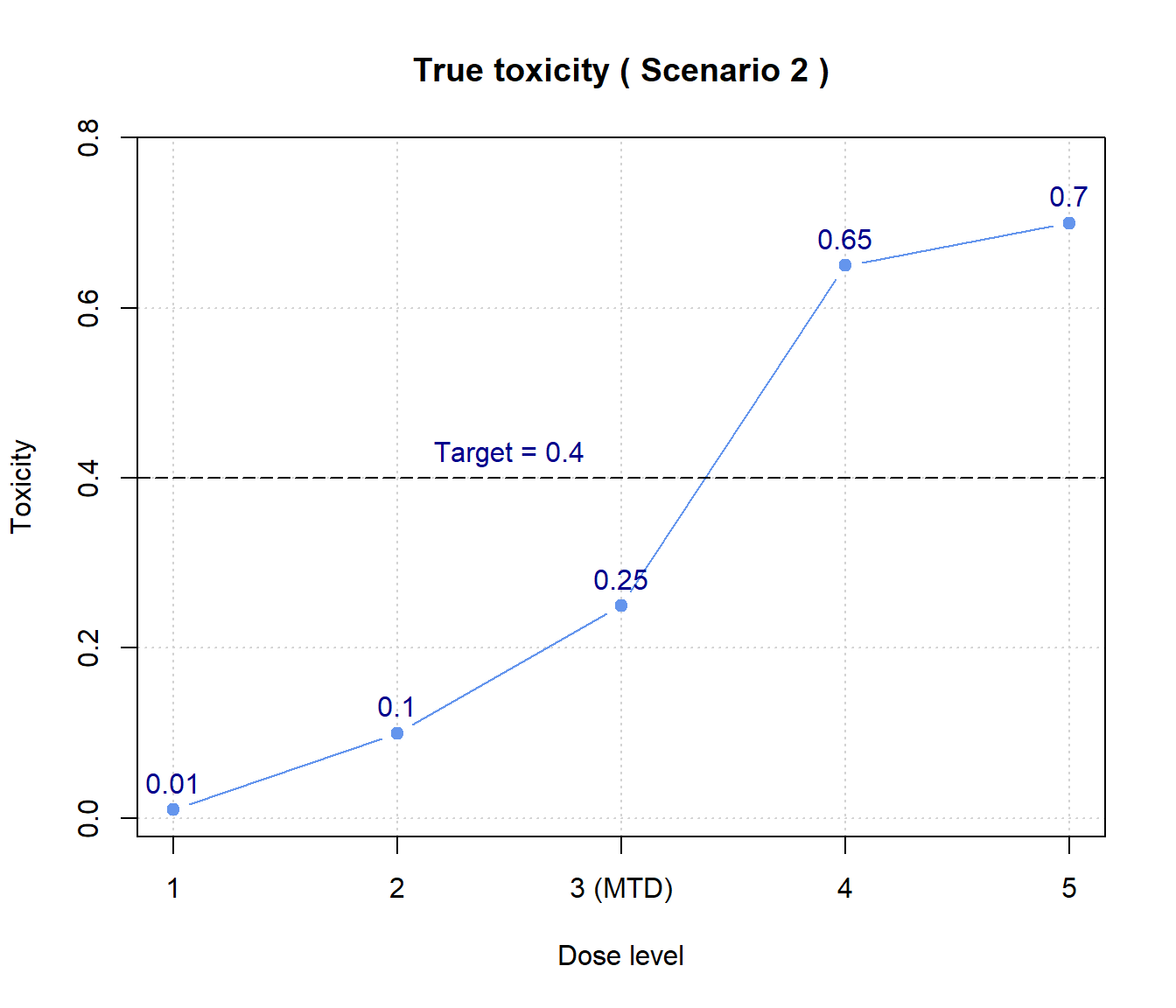# probability of selecting as MTD at each dose level
plot(out2, s = 2, pt = c(0.3, 0.4), type = "prob")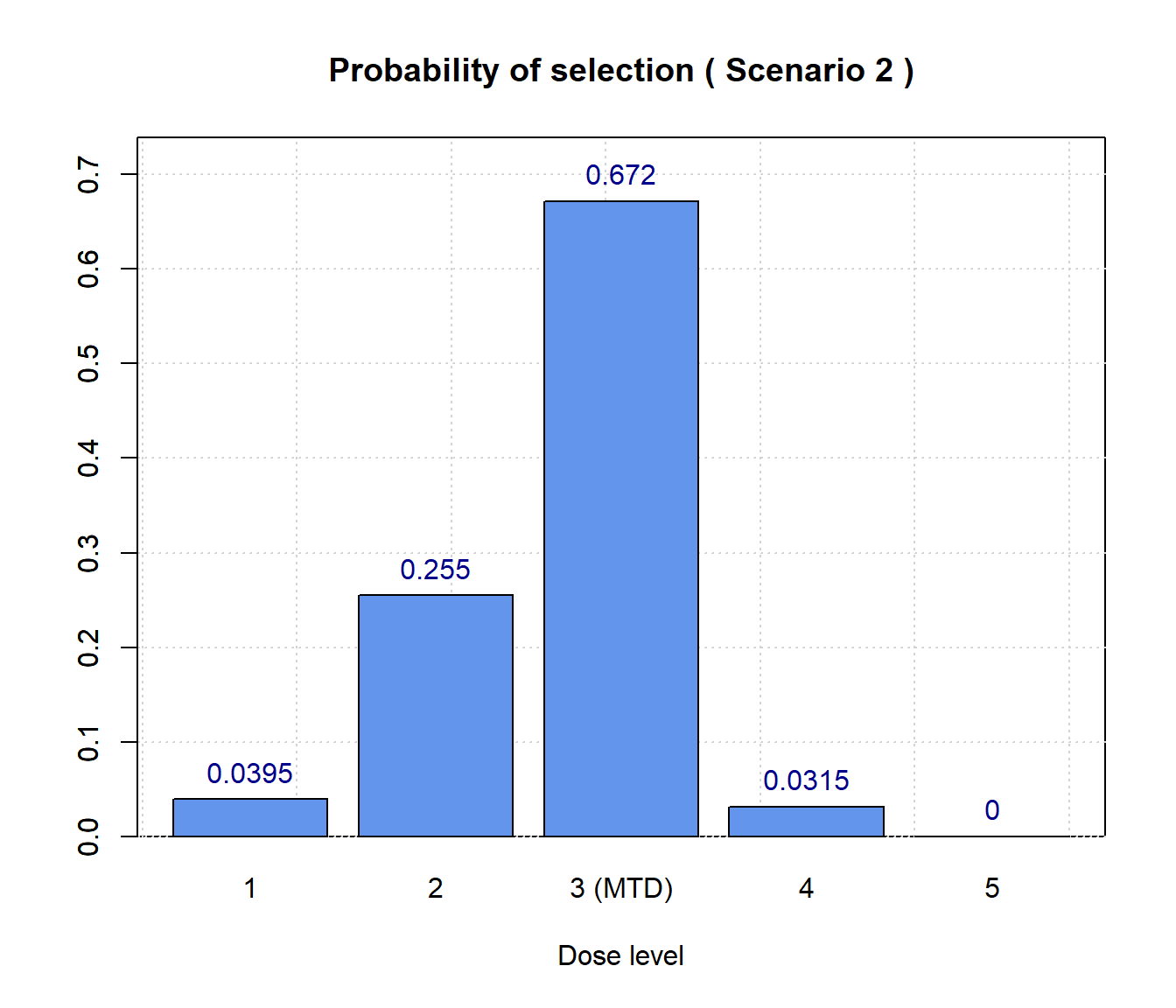# average number of patients treated at each dose level
plot(out2, s = 2, pt = c(0.3, 0.4), type = "np")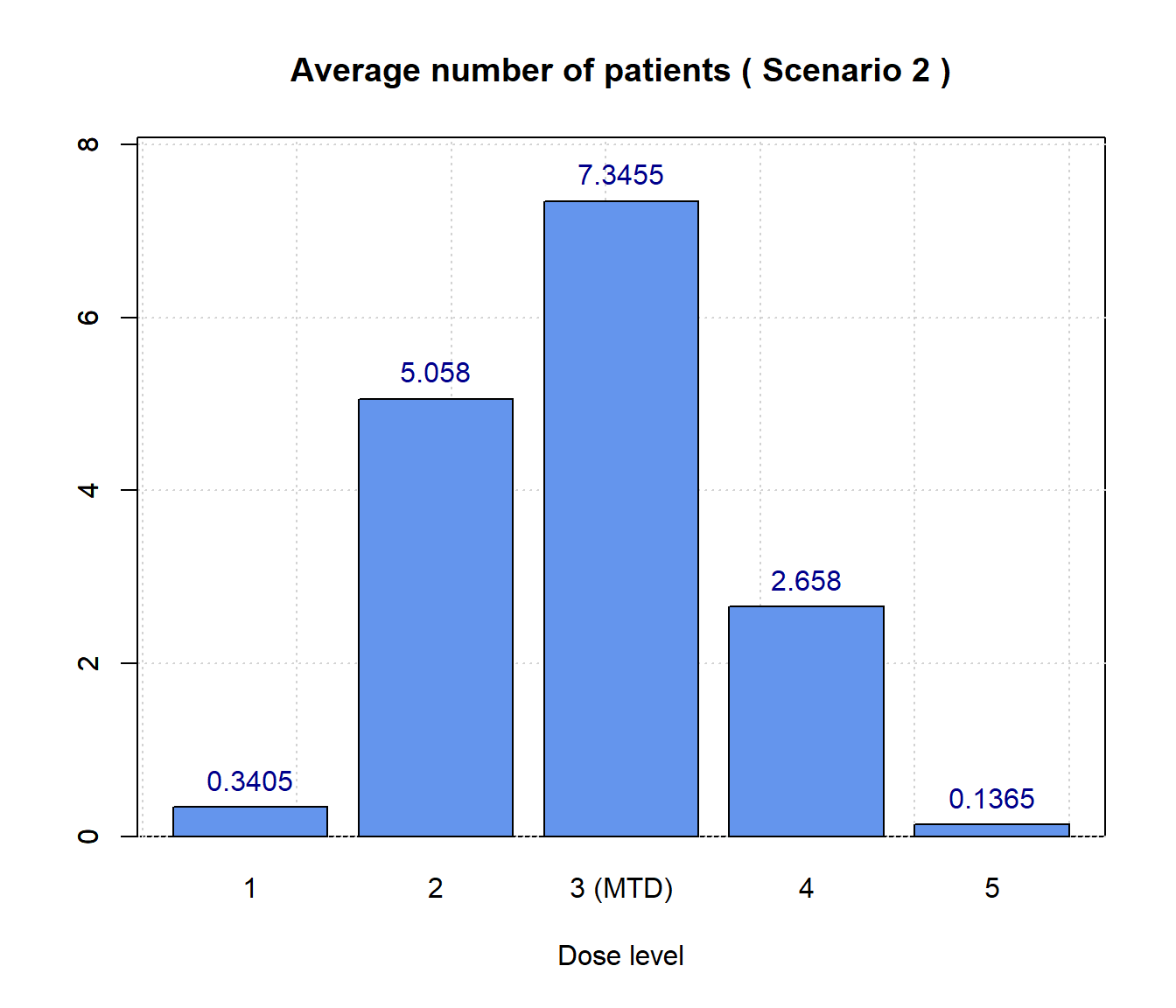# number of DLTs experienced at each dose level
plot(out2, s = 2, pt = c(0.3, 0.4), type="dlt")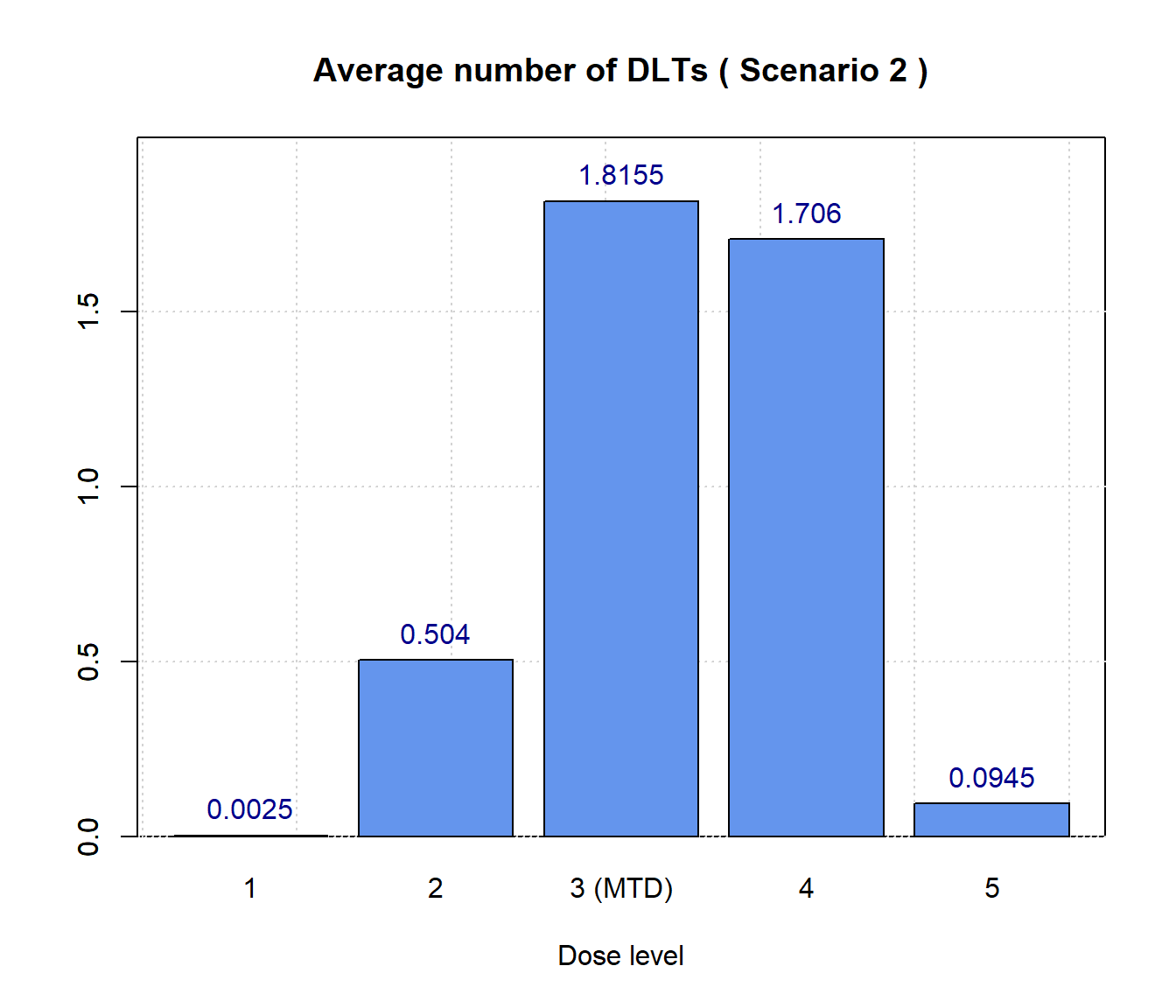There is a built-in function to put all different plots on same figure, simply run

plot(out2, pt = c(0.3, 0.4), type = "all", cex = 0.7)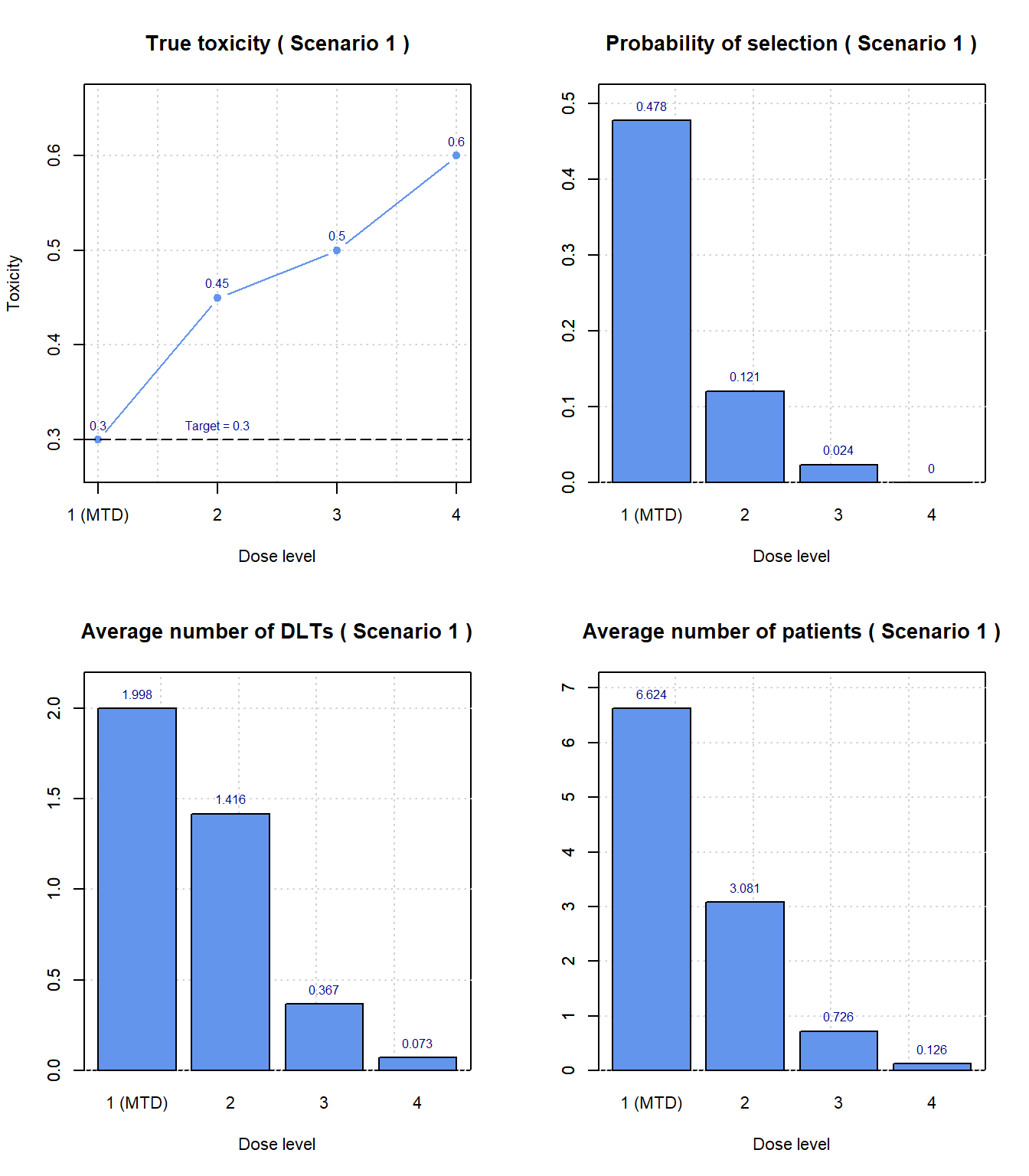## Scenarios list/customized table format

The .csv or .txt files used for sl.sim look like :

Start.Dose N.Trials Dose1 Dose2 Dose3 Dose4 Dose5
1 1000 0.30 0.45 0.50 0.60 NA
2 2000 0.01 0.10 0.25 0.65 0.7

This example is saved under \inst\extdata\testS.csv, use system.file to load it in R. The following variables have to be included: Start.dose : the starting dose level; N.trails : the number of simulated trials; Dose* : true probabilities of toxicity at the dose levels. Note that users don’t need put NA in the raw data if scenarios don’t have equal number of dose levels. In the above example, the NA on the first row is blank in the raw .csv file.

Both dec.sim and sl.sim support user-supplied decision table. It can be either matrix, data.frame or table in R. There is no checking on the format of the table in tsdf, so users need to make sure the input is a legitimate decision table for dose-finding. Also, the column name of the table should be the actual sample size instead of the default output of read.table which has column names X.* or V.*. For example, the following command reads the sample decision table in the package :

table.file <- system.file("extdata", "decTable.csv", package = "tsdf")
dec <- read.table(table.file, sep = ",", col.names = c(3,4,8,10), row.names = 1, check.names = FALSE)
colnames(dec)
##  "3"  "4"  "8"  "10"

Although there are many ways to import a decision table from .csv or .txt files, a typical decision table used for dev.sim and sl.sim functions have to have the following format when it’s loaded in R:

3 4 8 10
0 E E E E
1 S S E E
2 D D S E
3 DU DU D S
4 DU D D
5 DU D
6 DU DU
7 DU DU
8 DU DU
9 DU
10 DU